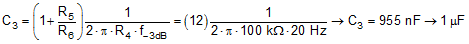SBOS566C June   2017  – October 2018

PRODUCTION DATA.

1. Features
2. Applications
3. Description
1.     Device Images
4. Revision History
5. Pin Configuration and Functions
6. Specifications
7. Detailed Description
1. 7.1 Overview
2. 7.2 Functional Block Diagram
3. 7.3 Feature Description
4. 7.4 Device Functional Modes
8. Application and Implementation
1. 8.1 Application Information
2. 8.2 Typical Application
3. 8.3 Other Application Examples
9. Power Supply Recommendations
10. 10Layout
11. 11Device and Documentation Support
12. 12Mechanical, Packaging, and Orderable Information

• D|8
• DGK|8
• DGK|8

#### 8.2.2 Detailed Design Procedure

The selected design requirements represent a high-performance wireless microphone application. Wireless microphones typically use an electret microphone element, an analog pre-amplifier circuit, and transmit circuitry which may use analog or digital methods of transmission. Because these devices are battery-powered, all circuitry must be designed to consume as little power as possible, while still achieving very high audio performance. The performance specifications for the microphone used in this design are shown in Table 2. This microphone element uses a 3-wire connection scheme with separate connections for power, ground, and signal. The microphone data sheet specifies that the signal line is terminated with a recommended 5.6-kΩ resistance and a 5-V supply.

### Table 2. Primo EM-173 Microphone Specifications

PARAMETER VALUE
Sensitivity –37 dBV
Output impedance 600 Ω
Signal-to-noise ratio (SNR) 80 dB
Maximum input sound pressure level 135 dB
Operating voltage 5 V (3 V – 10 V)
Operating current 600 µA

R1, C1, and R2 provide the correct termination impedance for the microphone and AC-couple the microphone signal to the amplifier input. R2 is selected with a large value (100 kΩ) so that a smaller AC-coupling capacitor can be used (C1). The high-pass corner frequency produced by C1 and R2 must be set to 20 Hz using Equation 1:

Equation 1.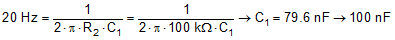R1 and R2 are in parallel for frequencies above 20 Hz. Therefore, select the value of R1 so that when in parallel with R2, the combination results in a 5.6-kΩ resistance as specified in the microphone data sheet. Equation 2 calculates R1.

Equation 2.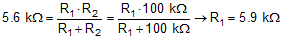R3 and C2 form a low-pass filter to prevent the amplification of electromagnetic interference (EMI) signals. Equation 3 shows the corner frequency of this EMI filter.

Equation 3.The input bias current of the OPA1692 through the 100-kΩ input resistor (R2) and can potentially cause a large offset voltage to appear at the output of the amplifier. One solution to this problem is to match the DC resistance of the circuit at each input of the amplifier. R4 and C3 accomplish this goal by providing a DC-feedback path for the amplifier (R4) which has the same resistance as the input resistor (R2). Capacitor C3 serves two functions. First, at low-frequencies this capacitor is effectively an open circuit and therefore the gain of the amplifier is 1, which reduces DC offsets at the output. At high frequencies where the impedance of the capacitor is low, the feedback network of R5, R6, and C4 determine the gain of the amplifier.

The nominal gain of the preamplifier circuit is calculated by considering the output of the microphone at the maximum input SPL. For this design, a maximum input SPL of 120 dB or [20 pascals (Pa)] is specified. The microphone sensitivity is shown as –37 dBV, measured at 1-Pa air pressure. The output signal of the microphone at 20-Pa air pressure can be calculated by converting the –37 dBV sensitivity specification to mV per pascal of air pressure as shown in Equation 4:

Equation 4.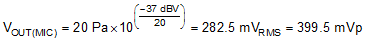The linear output voltage range of the OPA1692 extends to within 200 mV of each power supply. Therefore, on a ±5-V power supply, the linear output voltage range is ±4.8 V. The linear output voltage range of the amplifier and the maximum output signal level of the microphone determine the gain of the amplifier, as shown in Equation 5:

Equation 5.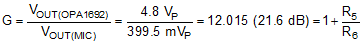Selecting values of 1.1 kΩ and 100 Ω for R5 and R6, respectively, produce a nominal gain of 12 for the circuit, allowing the full linear output swing of the amplifier to be used for the maximum input SPL. The feedback capacitor (C4) limits the gain of the circuit at high frequencies beyond the range of human hearing. Equation 6 shows the high-pass corner frequency that capacitor C4 produces:

Equation 6.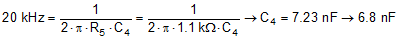Lastly, by the low-frequency bandwidth requirement for the design and the gain determines the value of C3. The high-pass corner frequency produced by this capacitor is affected by resistors R5 and R6 as shown in Equation 7:

Equation 7.